# Probabilistic Interpretations of Regression¶

## Gaussian Process Road Show, Genoa, Italy¶

### Overdetermined Systems¶

We motivated the introduction of probability by considering systems where there were more observations than unknowns. In particular we thought about the simple fitting of the gradient and an offset of a line,

$$y= mx +c$$

and what happens if we have three pairs of observations of $x$ and $y$, $\{x_i, y_i\}_{i=1}^3$. We solved this issue by introducing a type of slack variable, $\epsilon_i$, known as noise, such that for each observation we had the equation,

$$y_i = mx_i + c + \epsilon_i.$$

The slack variable represented the difference between our actual prediction and the true observation. This is also known as the residual. By introducing the slack variable we now have an additional $n$ variables to estimate, one for each data point, $\{\epsilon_i\}$. This actually turns the overdetermined system into an underdetermined system. Introduction of $n$ variables, plus the original $m$ and $c$ gives us $n+2$ parameters to be estimated from $n$ observations, which actually makes the system underdetermined. However, we then made a probabilistic assumption about the slack variables, we assumed that the slack variables were distributed according to a probability density. And for the moment we have been assuming that density was the Gaussian,

$$\epsilon_i \sim \mathcal{N}(0, \sigma^2),$$

with zero mean and variance $\sigma^2$.

#### Sum of Squares and Probability¶

In the overdetermined system we introduced a new set of slack variables, $\{\epsilon_i\}_{i=1}^n$, on top of our parameters $m$ and $c$. We deal with the variables by placing a probability distribution over them. This gives rise to the likelihood and for the case of Gaussian distributed variables, it gives rise to the sum of squares error. It was Gauss who first made this connection in his volume on "Theoria Motus Corprum Coelestium" (written in Latin)

In :
import pods

/Users/neil/Library/Enthought/Canopy_64bit/User/lib/python2.7/site-packages/pytz/__init__.py:29: UserWarning: Module pods was already imported from /Users/neil/sods/ods/pods/__init__.pyc, but /Users/neil/Library/Enthought/Canopy_64bit/User/lib/python2.7/site-packages is being added to sys.path
from pkg_resources import resource_stream


The relevant section roughly translates as

... It is clear, that for the product $\Omega = h^\mu \pi ^{-frac{1}{2}\mu} e^{-hh(vv + v^\prime v^\prime + v^{\prime\prime} v^{\prime\prime} + \dots)}$ to be maximised the sum $vv + v ^\prime v^\prime + v^{\prime\prime} v^{\prime\prime} + \text{etc}.$ ought to be minimized. Therefore, the most probable values of the unknown quantities $p , q, r , s \text{etc}.$, should be that in which the sum of the squares of the differences between the functions $V, V^\prime, V^{\prime\prime} \text{etc}$, and the observed values is minimized, for all observations of the same degree of precision is presumed.

It's on the strength of this paragraph that the density is known as the Gaussian, despite the fact that four pages later Gauss credits the necessary integral for the density to Laplace, and it was also Laplace that did a lot of the original work on dealing with these errors through probability. Stephen Stigler's book on the measurement of uncertainty before 1900 has a nice chapter on this.

In :
pods.notebook.display_google_book(id='ORUOAAAAQAAJ', page='217')

In :
%matplotlib inline
import numpy as np
import matplotlib.pyplot as plt
import pods
from IPython.display import display


Here numpy gives us the numerical array facility, matplotlib is for plotting and pods is a python open data science library which provides some convenient access to a range of data sets.

## Iterative Solution¶

Now we will code linear regression in python. We will do it in two ways, once using iterative updates (coordinate ascent) and then using linear algebra. For this part we are going to load in some data, we will use the example from the Olympics: the pace of Marathon winners. To load in the data we are going to use the pods library, an 'open data science' library for access to a range of data sets and with other facilities.

In :
data = pods.datasets.olympic_marathon_men()
x = data['X']
y = data['Y']


You can see what these values are by typing:

In :
print(x)
print(y)

[[ 1896.]
[ 1900.]
[ 1904.]
[ 1908.]
[ 1912.]
[ 1920.]
[ 1924.]
[ 1928.]
[ 1932.]
[ 1936.]
[ 1948.]
[ 1952.]
[ 1956.]
[ 1960.]
[ 1964.]
[ 1968.]
[ 1972.]
[ 1976.]
[ 1980.]
[ 1984.]
[ 1988.]
[ 1992.]
[ 1996.]
[ 2000.]
[ 2004.]
[ 2008.]
[ 2012.]]
[[ 4.47083333]
[ 4.46472926]
[ 5.22208333]
[ 4.15467867]
[ 3.90331675]
[ 3.56951267]
[ 3.82454477]
[ 3.62483707]
[ 3.59284275]
[ 3.53880792]
[ 3.67010309]
[ 3.39029111]
[ 3.43642612]
[ 3.20583007]
[ 3.13275665]
[ 3.32819844]
[ 3.13583758]
[ 3.0789588 ]
[ 3.10581822]
[ 3.06552909]
[ 3.09357349]
[ 3.16111704]
[ 3.14255244]
[ 3.08527867]
[ 3.10265829]
[ 2.99877553]
[ 3.03392977]]


## Plotting the Data¶

And you can make a plot of $y$ vs $x$ with the following command:

In :
plt.plot(x, y, 'rx')

Out:
[<matplotlib.lines.Line2D at 0x10dc0fc10>]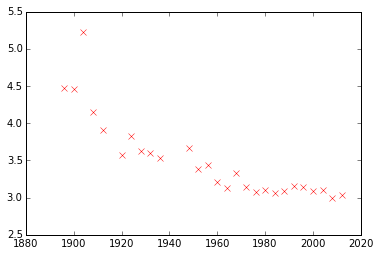## Maximum Likelihood: Iterative Solution¶

Now we are going to fit a line, $y_i=mx_i + c$, to the data you've plotted. We are trying to minimize the error function:

$$E(m, c, \sigma^2) = \frac{n}{2} \log \sigma^2 + \frac{1}{2\sigma^2} \sum_{i=1}^n(y_i-mx_i-c)^2$$

with respect to $m$, $c$ and $\sigma^2$. We can start with an initial guess for $m$,

In :
m = -0.4
c = 80


Then we use the maximum likelihood update, derived in the lecture, to find an estimate for the offset, $c$,

$$c^* = \frac{\sum_{i=1}^n(y_i-m^*x_i)}{n}$$
In :
c = (y - m*x).mean()
print c

786.019771145


And now we can make an estimate for the gradient of the line,

$$m^* = \frac{\sum_{i=1}^n ((y_i - c)*x_i))}{\sum_{i=1}^n x_i^2}$$
In :
m = ((y - c)*x).sum()/(x**2).sum()
print m

-0.3998724073


We can have a look at how good our fit is by computing the prediction across the input space. First create a vector of 'test points',

In :
x_test = np.linspace(1890, 2020, 130)[:, None]


Now use this vector to compute some test predictions,

In :
f_test = m*x_test + c


Now plot those test predictions with a blue line on the same plot as the data,

In :
plt.plot(x_test, f_test, 'b-')
plt.plot(x, y, 'rx')

Out:
[<matplotlib.lines.Line2D at 0x10dc18590>]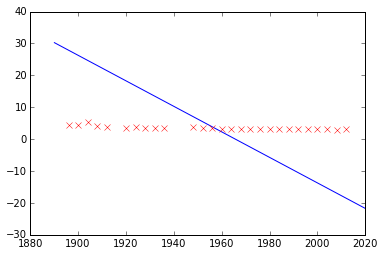The fit isn't very good, we need to iterate between these parameter updates in a loop to improve the fit, we have to do this several times,

In :
for i in np.arange(10):
m = ((y - c)*x).sum()/(x*x).sum()
c = (y-m*x).sum()/y.shape
print(m)
print(c)

-0.398725964251
783.527379727


And let's try plotting the result again

In :
f_test = m*x_test + c
plt.plot(x_test, f_test, 'b-')
plt.plot(x, y, 'rx')

Out:
[<matplotlib.lines.Line2D at 0x10dc311d0>]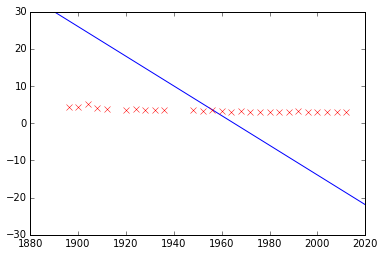Clearly we need more iterations than 10!

Try add more iterations above to try and get closer to the solution.

## Direct Solution with Linear Algebra¶

Hopefully, you are now persuaded of the merits of solving the entire system, simultaneously, using linear algebra. To do that, we need to make a design matrix of the data, which includes the $x_0=1$ column, to represent the bias, remember (from the lecture notes) that we are now moving to a system where our prediction is given by an inner product:

$$f(\mathbf{x}_i) = \mathbf{x}_i^\top\mathbf{w}$$

where each vector $\mathbf{x}_i$ is given by appending a 1 onto the original vector

$$\mathbf{x}_i = \begin{bmatrix} 1 \\\ x_i \end{bmatrix}$$

We can do this for the entire data set to form a design matrix $\mathbf{X}$,

$$\mathbf{X} = \begin{bmatrix} \mathbf{x}_1^\top \\\ \mathbf{x}_2^\top \\\ \vdots \\\ \mathbf{x}_N^\top \end{bmatrix} = \begin{bmatrix} 1 & x_1 \\\ 1 & x_2 \\\ \vdots & \vdots \\\ 1 & x_N \end{bmatrix},$$

which in numpy is done with the following commands:

In :
X = np.hstack((np.ones_like(x), x)) # [ones(size(x)) x]
print(X)

[[  1.00000000e+00   1.89600000e+03]
[  1.00000000e+00   1.90000000e+03]
[  1.00000000e+00   1.90400000e+03]
[  1.00000000e+00   1.90800000e+03]
[  1.00000000e+00   1.91200000e+03]
[  1.00000000e+00   1.92000000e+03]
[  1.00000000e+00   1.92400000e+03]
[  1.00000000e+00   1.92800000e+03]
[  1.00000000e+00   1.93200000e+03]
[  1.00000000e+00   1.93600000e+03]
[  1.00000000e+00   1.94800000e+03]
[  1.00000000e+00   1.95200000e+03]
[  1.00000000e+00   1.95600000e+03]
[  1.00000000e+00   1.96000000e+03]
[  1.00000000e+00   1.96400000e+03]
[  1.00000000e+00   1.96800000e+03]
[  1.00000000e+00   1.97200000e+03]
[  1.00000000e+00   1.97600000e+03]
[  1.00000000e+00   1.98000000e+03]
[  1.00000000e+00   1.98400000e+03]
[  1.00000000e+00   1.98800000e+03]
[  1.00000000e+00   1.99200000e+03]
[  1.00000000e+00   1.99600000e+03]
[  1.00000000e+00   2.00000000e+03]
[  1.00000000e+00   2.00400000e+03]
[  1.00000000e+00   2.00800000e+03]
[  1.00000000e+00   2.01200000e+03]]


From the multivariate regression solution we derived in the lecture, the maximum likelihood solution for $\mathbf{w}^*$ is given by

$$\mathbf{w}^* = \left[\mathbf{X}^\top \mathbf{X}\right]^{-1} \mathbf{X}^\top \mathbf{y}$$

First let's persuade ourselves of a few things. We suggested in the lecture that

$$\sum_{i=1}^n \mathbf{x}_i\mathbf{x}_i^\top = \mathbf{X}^\top\mathbf{X}$$

We can show that this is, indeed the case for our data. First we need to know how to do matrix multiplication and transpose using numpy, this is done as

In :
np.dot(X.T, X)

Out:
array([[  2.70000000e+01,   5.28200000e+04],
[  5.28200000e+04,   1.03365648e+08]])

Now we will compute the same thing with a for loop

In :
store = np.zeros((2, 2))
for i in range(X.shape):
store += np.outer(X[i, :], X[i, :])
print store

[[  2.70000000e+01   5.28200000e+04]
[  5.28200000e+04   1.03365648e+08]]


I hope that you agree that the first version is a little more compact.

### Solving the System¶

The solution for $\mathbf{w}$ is given in terms of a matrix inverse, but numerically this isn't the best way to compute it. What we actually want python to do is to solve the system of linear equations given by

$$\mathbf{X}^\top\mathbf{X} \mathbf{w} = \mathbf{X}^\top\mathbf{y}$$

for $\mathbf{w}$. This can be done in numpy using the command

In :
np.linalg.solve?


so we can obtain the solution using

In :
w = np.linalg.solve(np.dot(X.T, X), np.dot(X.T, y))
print w
print c
print m

[[  2.88952457e+01]
[ -1.29806477e-02]]
783.527379727
-0.398725964251


Allowing us to plot the fit as follows

In :
m = w; c=w
f_test = m*x_test + c
print(m)
print(c)
plt.plot(x_test, f_test, 'b-')
plt.plot(x, y, 'rx')

[-0.01298065]
[ 28.89524574]

Out:
[<matplotlib.lines.Line2D at 0x10dfbcdd0>]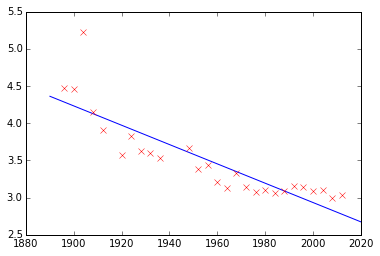## Introducing a Basis: Quadratic Fit¶

Now we will fit a quadratic model using basis functions. Given everything we've learnt above, this is now quite easy to do. Firstly, we need to create a design matrix that contains the quadratic basis,

$$\mathbf{\Phi} = \left[ \mathbf{1} \quad \mathbf{x} \quad \mathbf{x}^2\right]$$

where this notation means that each column of $\mathbf{\Phi}$ is derived from the entire set of input years.

In :
Phi = np.hstack([np.ones(x.shape), x, x**2])


Now we can solve this system for $\mathbf{w}$ just as we did for the linear case, so we have,

In :
w = np.linalg.solve(np.dot(Phi.T, Phi), np.dot(Phi.T, y))
print(w)

[[  6.43641954e+02]
[ -6.42502988e-01]
[  1.61109704e-04]]


We can plot the solution in two different ways, either we take

In :
f_test = w*x_test**2 + w*x_test + w
plt.plot(x_test, f_test, 'b-')
plt.plot(x, y, 'rx')

Out:
[<matplotlib.lines.Line2D at 0x10e381610>]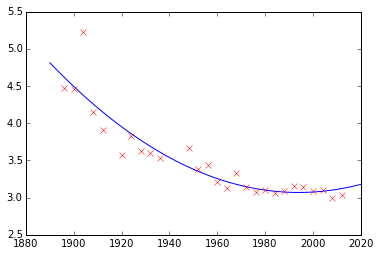Or we can do the matrix form of this equation which first involves creating a design matrix for the test points,

In :
Phi_test = np.hstack((np.ones_like(x_test), x_test, x_test**2))


and then computing the value of the function using a matrix multiply

In :
f_test = np.dot(Phi_test,w)
plt.plot(x_test, f_test, 'b-')
plt.plot(x, y, 'rx')
w

Out:
array([[  6.43641954e+02],
[ -6.42502988e-01],
[  1.61109704e-04]])Note the values of the coefficient $w_2$ in particular. It is relatively small, because it is multiplying a large number (square of 2000 is 4 million). This need to use small coefficients becomes worse as we increase the order of the fit. As an exercise for later, try fitting higher order polynomials to the data. See what happens as you increase the polynomial order.

## Generalization¶

The aim of this notebook is to review the different methods of model selection: hold out validation, leave one out cross validation and cross validation.

## Training Error¶

The first thing we'll do is plot the training error for the polynomial fit. To do this let's set up some parameters.

In :
num_data = x.shape
num_pred_data = 100 # how many points to use for plotting predictions
x_pred = np.linspace(1890, 2016, num_pred_data)[:, None] # input locations for predictions
order = 4 # The polynomial order to use.



now let's build the basis matrices.

In :
# build the basis set
Phi = np.zeros((num_data, order+1))
Phi_pred = np.zeros((num_pred_data, order+1))
for i in range(0, order+1):
Phi[:, i:i+1] = x**i
Phi_pred[:, i:i+1] = x_pred**i


now we can solve for the regression weights and make predictions both for the training data points, and the test data points. That involves solving the linear system given by

$$\boldsymbol{\Phi}^\top \boldsymbol{\Phi} \mathbf{w}^* = \boldsymbol{\Phi}^\top \mathbf{y}$$
In :
# solve the linear system
w_star = np.linalg.solve(np.dot(Phi.T, Phi), np.dot(Phi.T, y))


and using the resulting vector to make predictions at the training points and test points,

$$\mathbf{f} = \boldsymbol{\Phi}\mathbf{w}.$$

To implement this in practice we need to use basis matrices for both the predictions and the training points.

In :
# predict at training and test points
f = np.dot(Phi, w_star)
f_pred = np.dot(Phi_pred, w_star)


These can be used to compute the error

$$E(\mathbf{w}) = \frac{n}{2} \log \sigma^2 + \frac{1}{2\sigma^2} \sum_{i=1}^n \left(y_i - \mathbf{w}^\top \phi(\mathbf{x}_i)\right)^2 \\\ E(\mathbf{w}) = \frac{n}{2} \log \sigma^2 + \frac{1}{2\sigma^2} \sum_{i=1}^n \left(y_i - f_i\right)^2$$
In :
# compute the sum of squares term
sum_squares = ((y-f)**2).sum()
# fit the noise variance
sigma2 = sum_squares/num_data
error = 0.5*(num_data*np.log(sigma2) + sum_squares/sigma2)


Now we have the fit and the error, let's plot the fit and the error.

In :
# print the error and plot the predictions
print("The error is: %2.4f"%error)
plt.plot(x_pred, f_pred)
plt.plot(x, y, 'rx')
ax = plt.gca()
ax.set_title('Predictions for Order 5')
ax.set_xlabel('year')
ax.set_ylabel('pace (min/km)')

The error is: -29.9371

Out:
<matplotlib.text.Text at 0x10e723a10>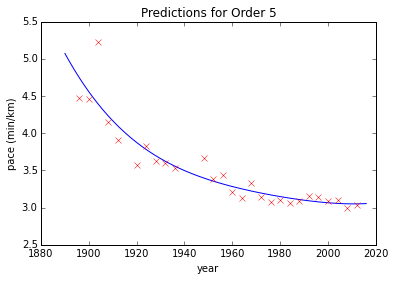# Solution with QR Decomposition¶

Performing a solve instead of a matrix inverse is the more numerically stable approach, but we can do even better. A QR-decomposition of a matrix factorises it into a matrix which is an orthogonal matrix $\mathbf{Q}$, so that $\mathbf{Q}^\top \mathbf{Q} = \mathbf{I}$. And a matrix which is upper triangular, $\mathbf{R}$. $$\boldsymbol{\Phi}^\top \boldsymbol{\Phi} \mathbf{w} = \boldsymbol{\Phi}^\top \mathbf{y}$$ $$(\mathbf{Q}\mathbf{R})^\top (\mathbf{Q}\mathbf{R})\mathbf{w} = (\mathbf{Q}\mathbf{R})^\top \mathbf{y}$$ $$\mathbf{R}^\top (\mathbf{Q}^\top \mathbf{Q}) \mathbf{R} \mathbf{w} = \mathbf{R}^\top \mathbf{Q}^\top \mathbf{y}$$ $$\mathbf{R}^\top \mathbf{R} \mathbf{w} = \mathbf{R}^\top \mathbf{Q}^\top \mathbf{y}$$ $$\mathbf{R} \mathbf{w} = \mathbf{Q}^\top \mathbf{y}$$ This is a more numerically stable solution because it removes the need to compute $\boldsymbol{\Phi}^\top\boldsymbol{\Phi}$ as an intermediate. Computing $\boldsymbol{\Phi}^\top\boldsymbol{\Phi}$ is a bad idea because it involves squaring all the elements of $\boldsymbol{\Phi}$ and thereby potentially reducing the numerical precision with which we can represent the solution. Operating on $\boldsymbol{\Phi}$ directly preserves the numerical precision of the model.

This can be more particularly seen when we begin to work with basis functions in the next session. Some systems that can be resolved with the QR decomposition can not be resolved by using solve directly.

In :
import scipy as sp
Q, R = np.linalg.qr(X)
w = sp.linalg.solve_triangular(R, np.dot(Q.T, y))
w

Out:
array([[  2.88952457e+01],
[ -1.29806477e-02]])

## Putting it Together¶

Now we look at how we can fit different polynomial basis sets to the olympic data and compute the training error.

In :
# import the time module to allow python to pause.
import time
# import the IPython display module to clear the output.
from IPython.display import clear_output, display

def polynomial(x, degree):
degrees = np.arange(degree+1)
return x**degrees

num_data = len(x)
error_list = []
max_degree = 6
sigma2 = 1
fig, axes = plt.subplots(nrows=1, ncols=2, figsize=(12,6))
for degree in range(0, max_degree+1):

# 1. build the basis set
Phi = polynomial(x, degree)
Phi_pred = polynomial(x_pred, degree)

# 2. solve the linear system with QR decomposition
Q, R = np.linalg.qr(Phi)
w = sp.linalg.solve_triangular(R, np.dot(Q.T, y))

# 3. make predictions at training and test points
f_pred = np.dot(Phi_pred, w)
f = np.dot(Phi, w)

# 4. compute the error and append it to a list.
error_list.append(((y-f)**2).sum() + num_data/2.*np.log(sigma2))

# 5. plot the predictions
axes.clear()
axes.clear()
axes.plot(x_pred, f_pred, linewidth=2)
axes.plot(x, y, 'rx', markersize=10, linewidth=2)
axes.set_ylim((2.5, 5.5))
axes.set_title('Predictions for Degree ' + str(degree) + ' model.')
axes.plot(np.arange(0, degree+1), np.asarray(error_list), linewidth=2)
axes.set_xlim((0, max_degree))
axes.set_ylim((0, 10))
axes.set_title('Training Error')
display(fig)
time.sleep(1)
clear_output()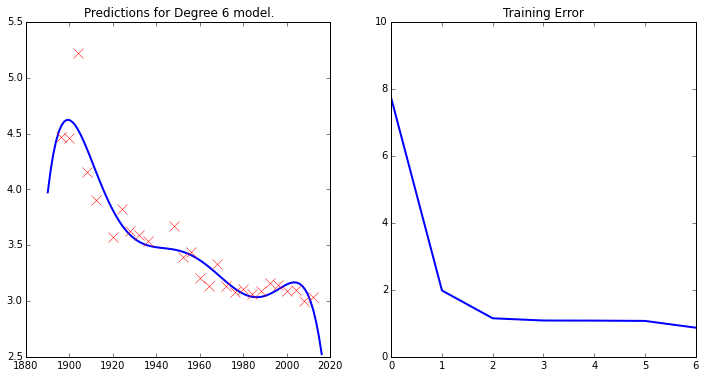In :